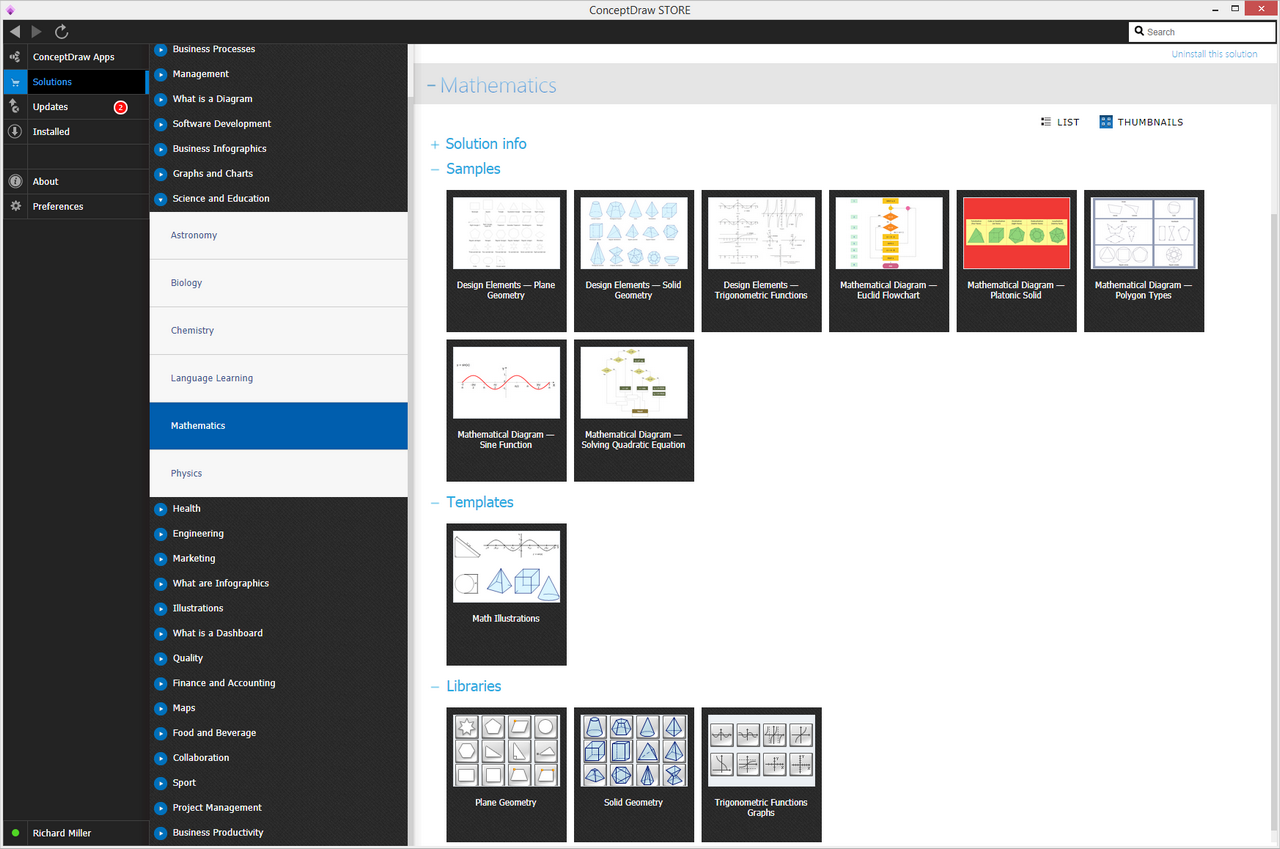This site uses cookies. By continuing to browse the ConceptDraw site you are agreeing to our Use of Site Cookies.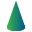# Mathematics

'Mathematics is the music of reason' — James Joseph Sylvester

Mathematics is the study of the following topics: structure space, quantity, and change. Seeking out different patterns and using them after for formulating some new conjectures, mathematicians resolve either the falsity or truth of conjectures by mathematical proof.

Once the mathematical structures are known to be good models of some real phenomena, then the so-called “mathematical reasoning” can provide predictions or an insight about nature. Through the use of logic and abstraction, mathematics developed from calculation, counting, the systematic study and the measurement of the motions and shapes of different physical objects. Being a human activity from as far back as written records exist, practical mathematics may be referred to a process of solving different mathematical problems.

Mathematics is simply essential in many fields, such as natural science, medicine, finance, the social sciences, and engineering. Mathematicians often face the need for creating the mathematical drawings in order to explain different theories and equations. In order to make such illustrations, the ConceptDraw DIAGRAM diagramming and drawing software may be used.

The Mathematics solution can be used while working in the ConceptDraw DIAGRAM application using its pre-made samples and templates as well as vector shape libraries of both solid and plane geometric figures, trigonometrical functions and mathematical symbols. It may help many mathematicians to create numeral mathematical diagrams, mathematic illustrations and tape diagrams for either scientific or educational, or both purposes.

There are 3 stencil libraries containing 50 vector symbols in the Mathematics solution.

## Mathematics Symbols — Solid Geometry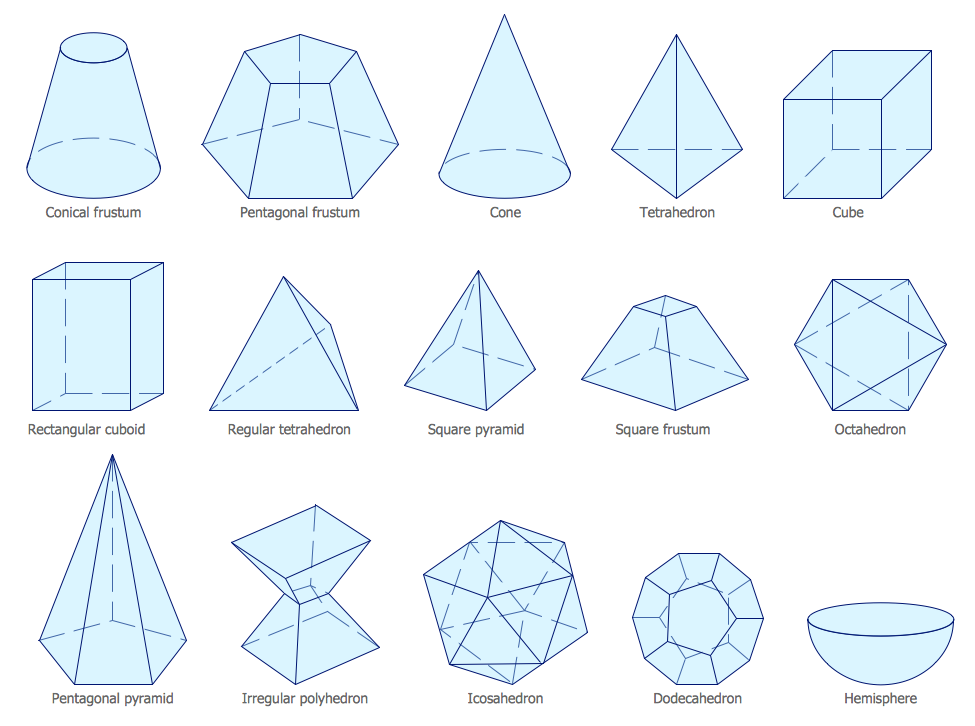## Mathematics Symbols — Trigonometric Functions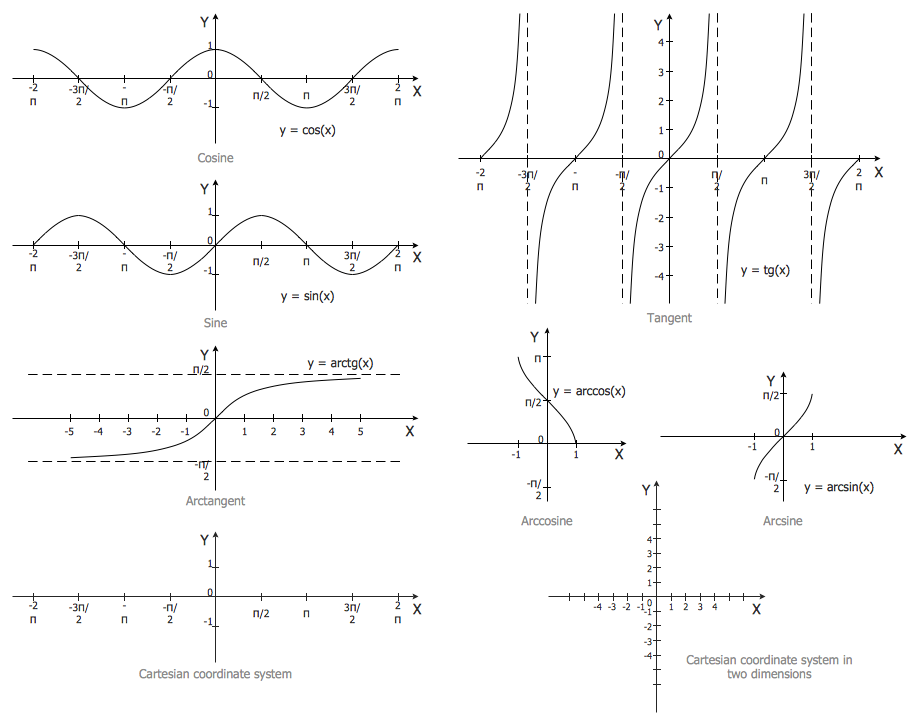## Examples

There are a few samples that you see on this page which were created in the ConceptDraw DIAGRAM application by using the Mathematics solution. Some of the solution's capabilities as well as the professional results which you can achieve are all demonstrated here on this page.

All source documents are vector graphic documents which are always available for modifying, reviewing and/or converting to many different formats, such as MS PowerPoint, PDF file, MS Visio, and many other graphic ones from the ConceptDraw Solution Park or ConceptDraw STORE. The Mathematics solution is available to all ConceptDraw DIAGRAM users to get installed and used while working in the ConceptDraw DIAGRAM diagramming and drawing software.

## Example 1: Mathematical Diagram — Solving Quadratic Equation

This diagram was created in ConceptDraw DIAGRAM using the Plane Geometry Library from the Mathematics Solution. An experienced user spent 10 minutes creating this sample.

This sample shows the flowchart of an algorithm that can solve quadric equations. It’s a useful and convenient way to represent different algorithms in the form of flowcharts.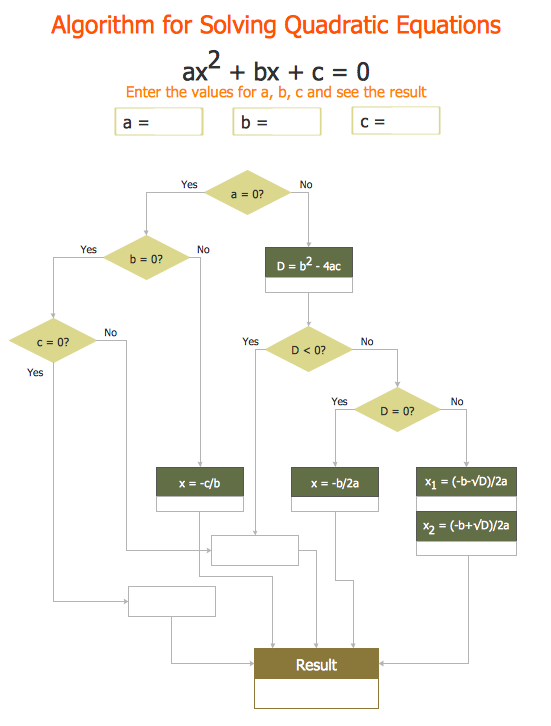## Example 2: Mathematical Diagram — Euclid Flowchart

This diagram was created in ConceptDraw DIAGRAM using the Plane Geometry Library from the Mathematics Solution. An experienced user spent 10 minutes creating this sample.

This sample demonstrates a flowchart depicting the Euclid's algorithm. The Greek mathemetician Euclid first described this around 300BC; now using the Mathematics Solution for ConceptDraw DIAGRAM, you can bring this and many other mathematical algorithms, mathematical diagrams, or tape diagrams to your computer screen quickly and easily.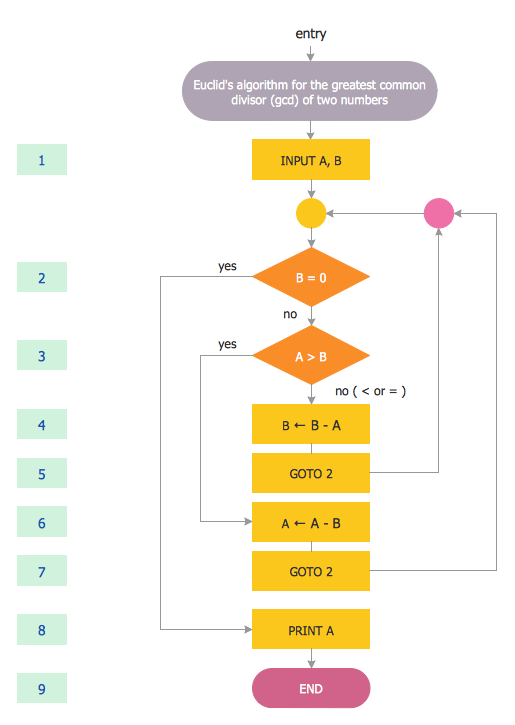## Example 3: Mathematical Diagram — Platonic Solid

This diagram was created in ConceptDraw DIAGRAM using the Solid Geometry Library from the Mathematics Solution. An experienced user spent 5 minutes creating this sample.

This sample visualizes the 5 platonic solids. A platonic solid is a convex regular polyhedron that consists of identical regular polygons and which has spatial symmetry. All these complex vector shapes were designed and included in the Solid Geometry Library.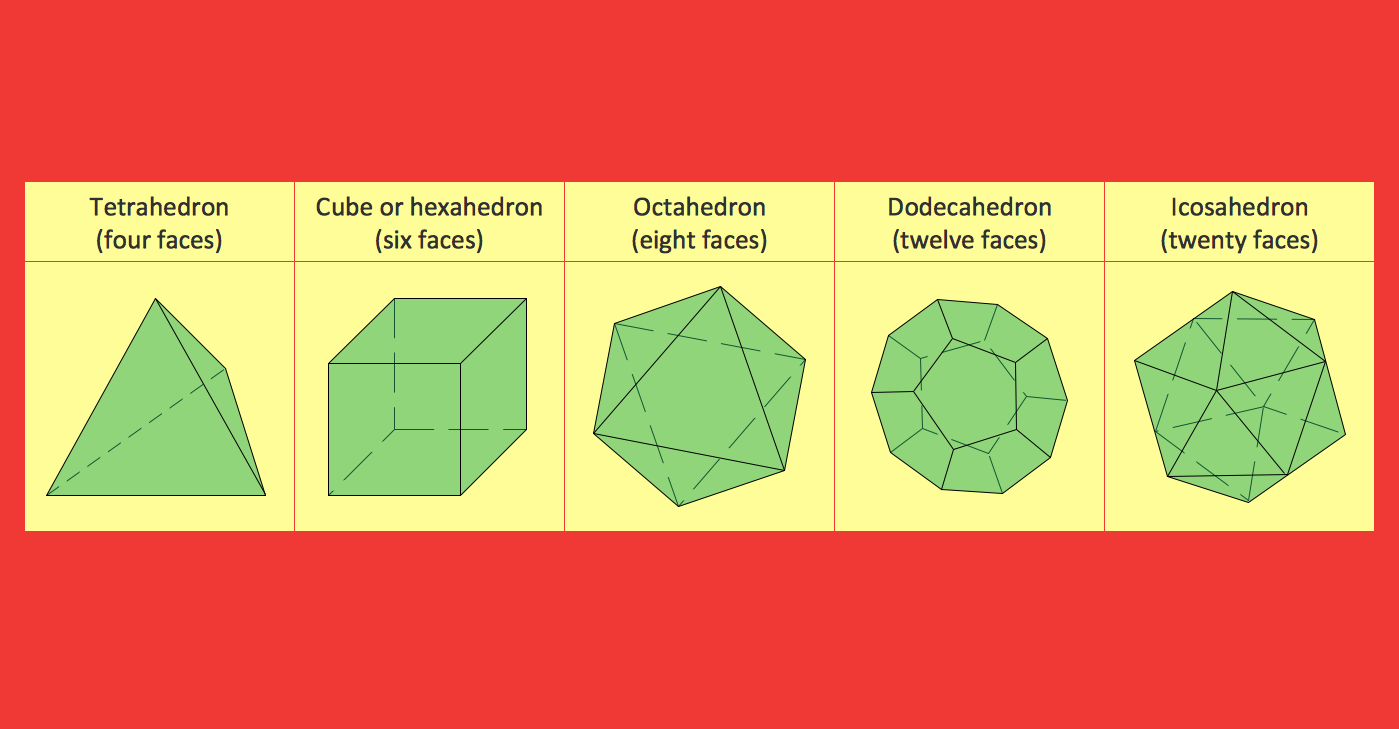## Example 4: Mathematical Diagram — Polygon Types

This diagram was created in ConceptDraw DIAGRAM using the Plane Geometry Library from the Mathematics Solution. An experienced user spent 5 minutes creating this sample.

This sample shows various polygon types. By utilising predesigned plane and solid geometric figures, mathematical symbols from the Mathematics Solution libraries help you save time when creating mathematical diagrams .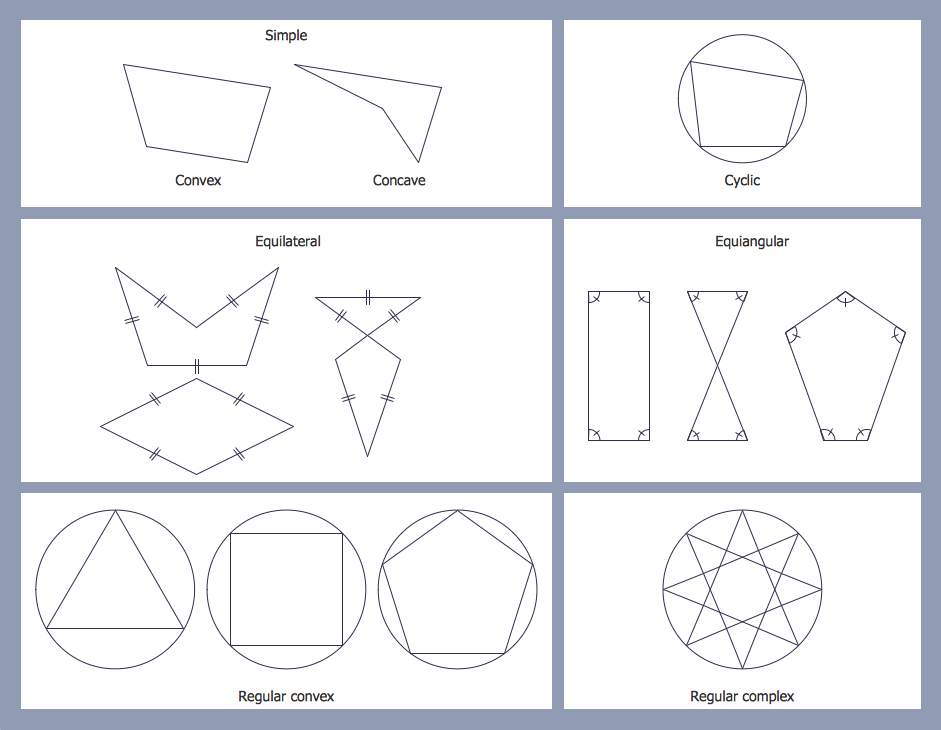## Example 5: Mathematical Diagram — Sine Function

This diagram was created in ConceptDraw DIAGRAM using the Trigonometric Functions Library from the Mathematics Solution. An experienced user spent 5 minutes creating this sample.

This sample illustrates the graph of the sine function. Sine is one of the basic trigonometric functions of an angle which is calculated as the ratio of opposite side to the hypotenuse. The Trigonometric Functions Library provides you with the predesigned graphs for all basic trigonometric functions.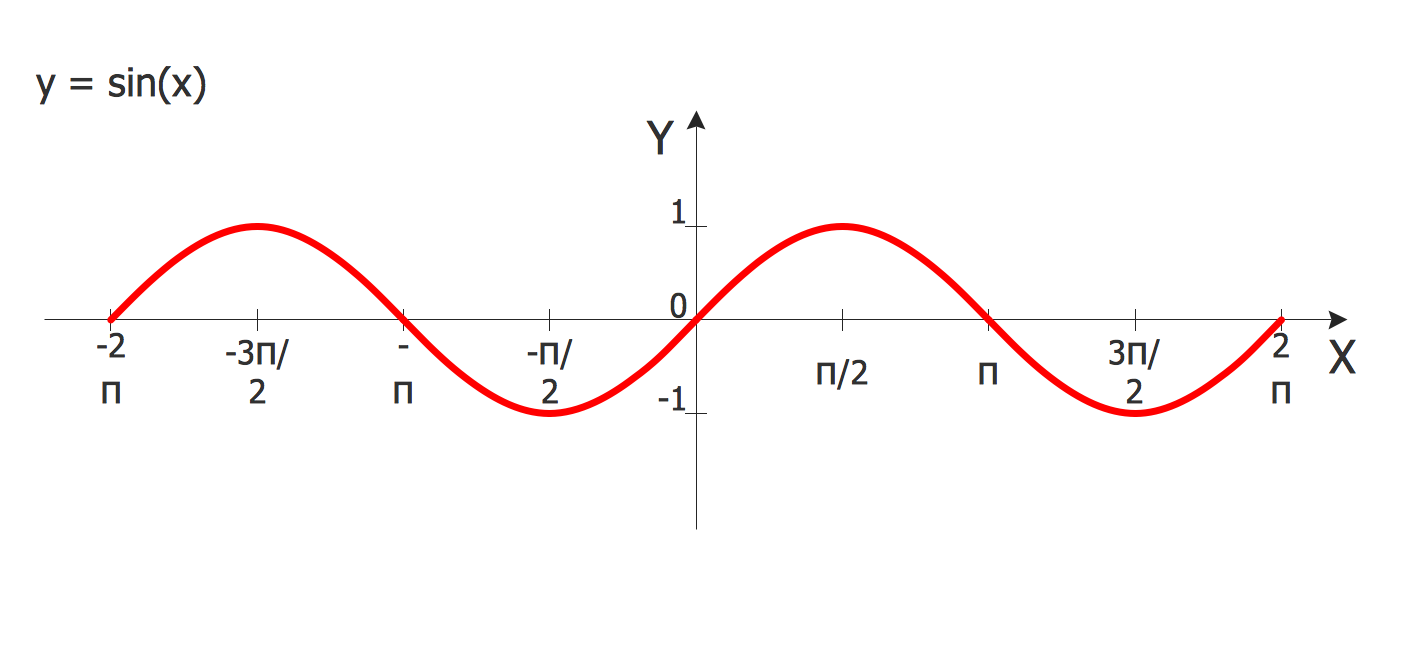# Video

00:00:00
00:00:00

## Inside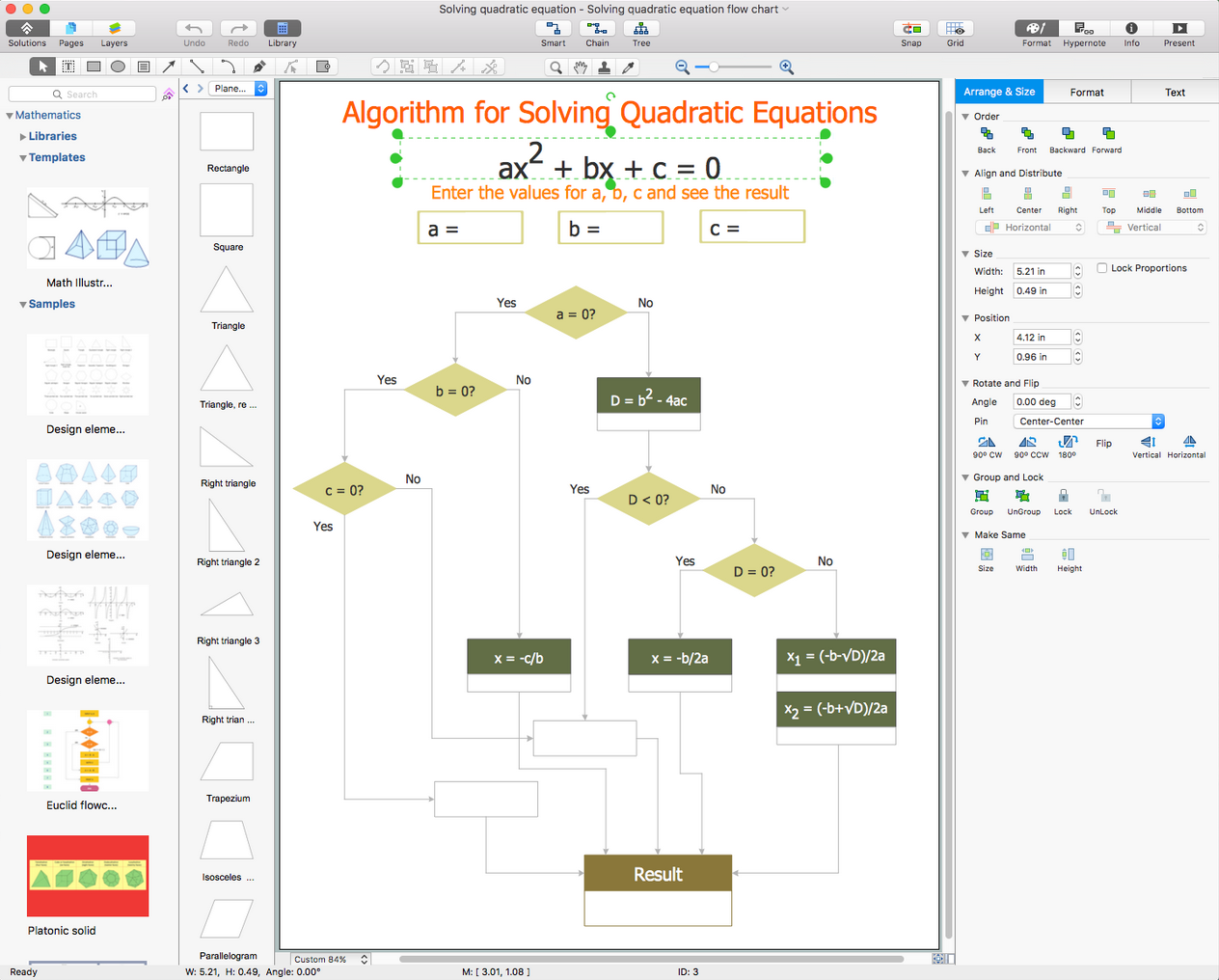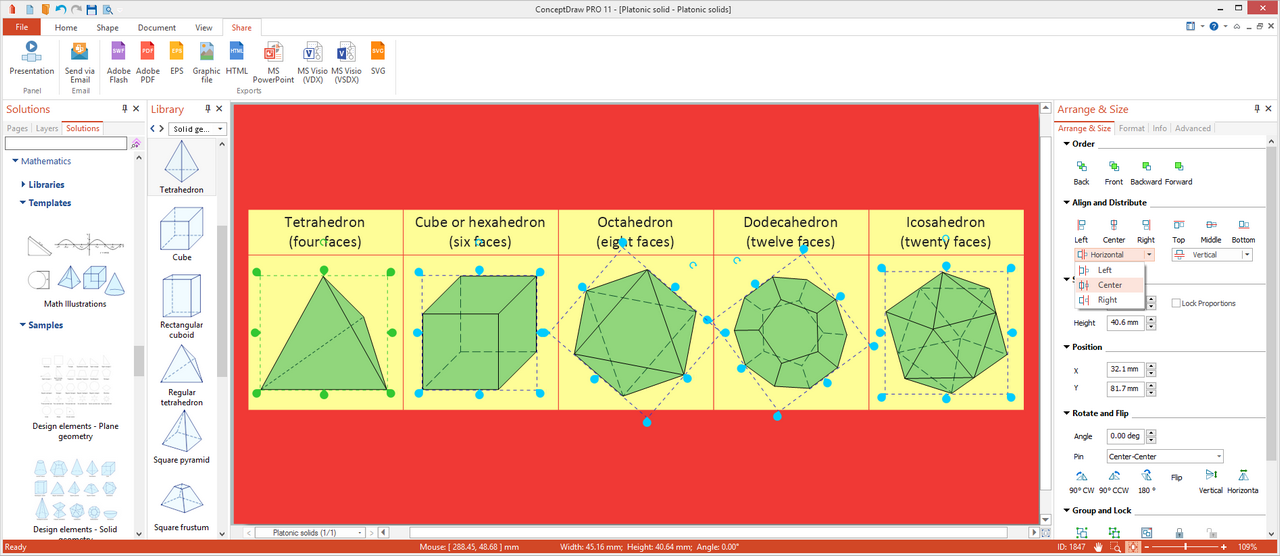## What I Need to Get Started

Both ConceptDraw DIAGRAM diagramming and drawing software and the Mathematics solution can help creating the learning materials you need. The Mathematics solution can be found in the Science and Education area of ConceptDraw STORE application that can be downloaded from this site. Make sure that both ConceptDraw DIAGRAM and ConceptDraw STORE applications are installed on your computer before you get started.

#### How to install

After ConceptDraw STORE and ConceptDraw DIAGRAM are downloaded and installed, you can install the Mathematics solution from the ConceptDraw STORE.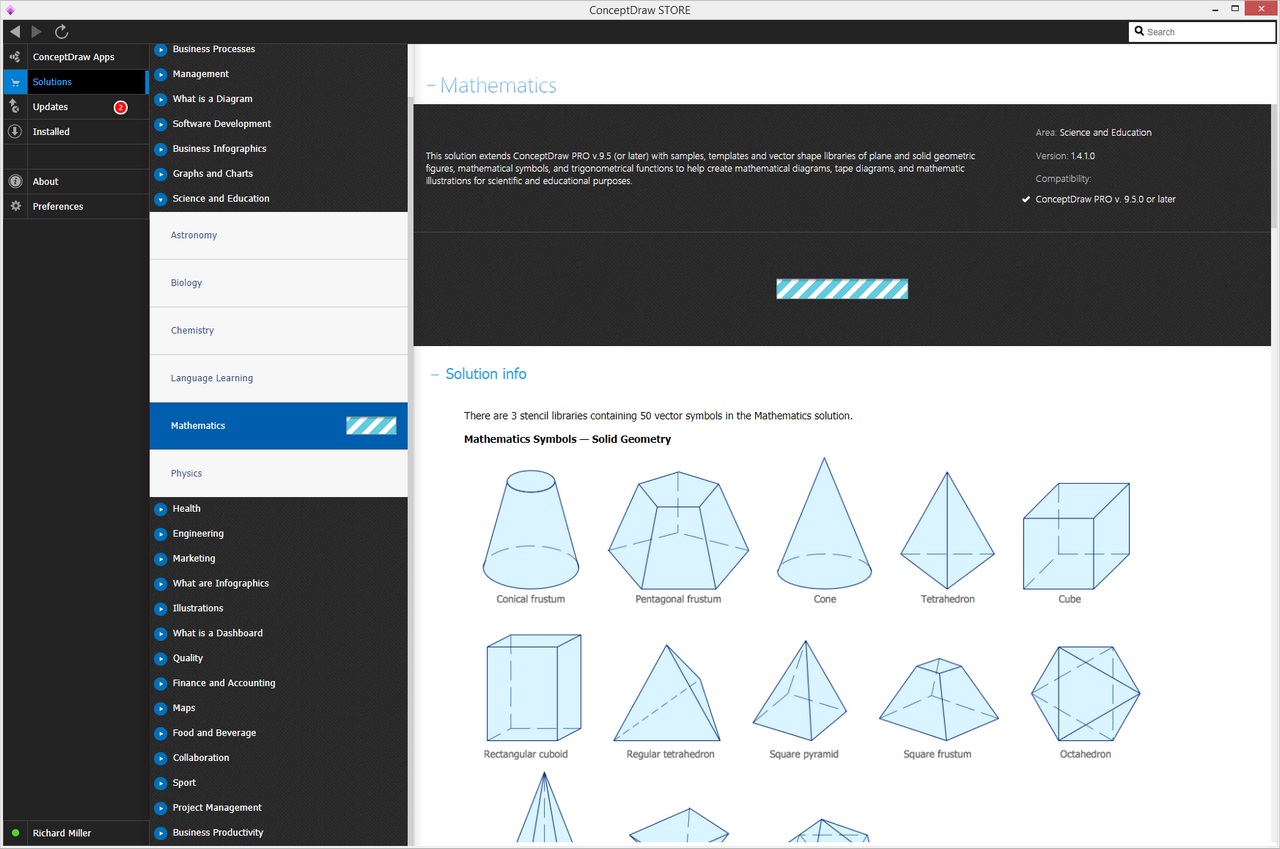#### Start Using

To make sure that you are doing it all right, use the pre-designed symbols from the stencil libraries from the solution to make your drawings look smart and professional. Also, the pre-made examples from this solution can be used as drafts so your own drawings can be based on them. Using the samples, you can always change their structures, colors and data.